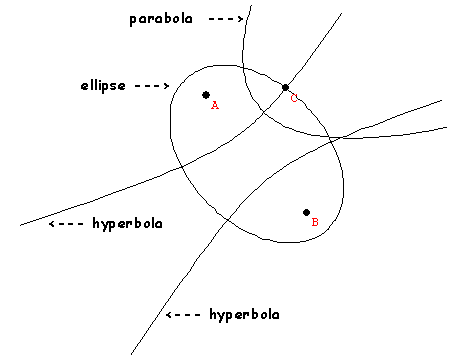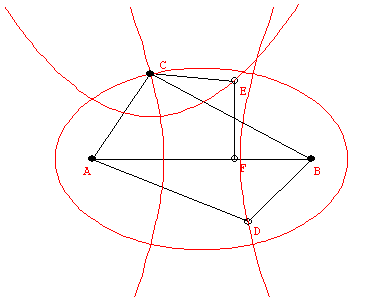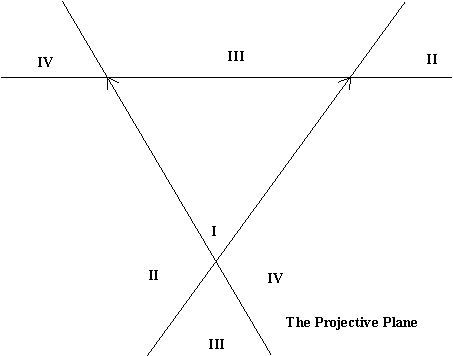Thursday,  November 6

Perpective from a point.... what happens to a circle in a plane?

The points on the circle and the center of perspective determine lines through the center.

The surface of these lines is a cone.

When you pass the cone through Flatland your see curves- the "conic sections".
Check out this applet.

These are....Google "conics."   much is found!   :)

Show video on Conics  FAPPThe characterization of points on a conic by measurements are:
ellipse:  AC + BC = constant
parabola: EC = EF
hyperbola: |CB-CA| = constant.
Rope Conic Activity.
Using a rope we formed a human ellipse.
After that we demonstrated how to use a rope to form a hyperbola and then a parabola.

String drawing conic activity.
End of class.

Conics Video.
Key background: Tangents to a circle are equal in length.

In  right triangle, the ratios of  the length of the side
opposite an angle to the hypotenuse length depends only on the angle. This ratio is called the sine of the angle and is abbreviated "sin".

Equations for conics in coordinate geometry.
Using coordinates to draw in perspective.

What do the conic look like in a perspective drawing or in the projective plane?